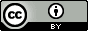## Monday, December 30, 2013

### Wolfram|Alpha and chemistry homework problems

Update 2014.01.20: This video really inspired this post and nicely addresses many of the objections one might raise.

About two weeks ago I wrote a blogpost wondering to what extent many chemistry homework problems are rendered trivial by chemical calculators.  As part of the post I started a little experiment where I see how many problems posted on Reddit's Chemhelp page I could solve using such calculators. I subsequently expanded the experiment to include Yahoo Answers chemistry page. Here you can see my contributions for Reddit and Yahoo Answers, but I'll focus on the Yahoo Answers.

As I am writing this I have supplied 21 answers on Yahoo Answers and 11 answers on Reddit (plus 1 in the mathhelp page). All but 3 of the answers involve Wolfram|Alpha (WA) so this is what I'll focus on here.  Most of these questions can be answered in two steps using WA and many can be answered in one step, i.e. one simply reformulates the question, types it in to WA and gets the answer.

Examples of the latter type of question:

"How many grams of sulfur (S) are needed to react completely with 246 g of mercury (Hg) to form mercuric sulfid?" which can be answered by typing the following into WA: "convert 246 g Hg to mercuric sulfid" (which also fixes the spelling).

"Calculate the number of moles of Al2O3 produced from 27.6 moles of O2" => "convert 27.6 moles O2 to Al2O3"

"How many grams of NaOH are in 250 mL of a 3.0 M NaOH solution?" => "convert 250 mL 3.0 M NaOH to grams"

"Na2CO3+Pt(ClO3)2 -> NaClO3 + PtCO3. Balance the equation.?" => "Na2CO3+Pt(ClO3)2 -> NaClO3 + PtCO3"

"C2H6+O2-> CO2+H2O. Draw Lewis dot structures for each species.?" => "lewis structure of CO2"

"Calculate the empirical formula of a molecule with the following percent compositions: 59.7% lithium (Li) and 40.3% nitrogen." => "59.7% lithium and 40.3% nitrogen"

"What is the number of mmoles solute in 2 liters of 0.0555 M KMnO?" => "convert 2 liters of 0.0555 M KMnO to mmoles"

"A 10% kcl solution in water has a density of 1.06/ml'calculate its malarity and malality?" => "convert 10 % KCl to malality"

"the blue color in some fireworks occurs when copper (I) chloride is heated to 1500K and emits a blue light of wavelength 4.50*10^2nm. How much energy does one photon of this light carry?" => "convert 485 nm wavelength to joules"

"What is the amount of substance of hydrogen atoms in 0,50g methane (CH4) and why?" => "how much hydrogen in 0.50 grams of methane"

"If 500 grams of Fe reacts with ample Oxygen according to the following equation: 4Fe + 3O2 = 2Fe2O3 A) How many grams of rust are produced? B) How many grams of Oxygen are needed?" => "how much Fe2O3 from 500 grams Fe"

These questions require a bit more knowledge:

"What mass of phosphorous is required to manufacture 2 metric tons (2000 kg) calcium phosphate?" => "convert 2000 kg calcium phosphate to phosphorous".  By default WA interprets calcium phosphate as hydroxyapatite but alerts you to the fact that tricalcium diphosphate is an alternative.  This is a poorly phrased question.
"E=200 M^ -1 Cm^ -1 A=1 Pathlength=0.5 cm Concentration of protein???" => "beer lambert equation"

"Balance the following redox reactionin basic solution using half reaction method?  O2 + Cr 3+ -> H2O2 + Cr2O7 2- to find how many electrons are lost and gained during the reaction" => "O2 + Cr^3+ + OH- -> H2O2 + Cr2O7^2- + e- + H2O" While not straightforward the equation doesn't balance in WA until OH-, e-, and H2O are put on the correct sides.  So this can really be solved by trial and error, once you know translate "basic solution" into these constituents.

"From 0.65 g CaClx, 1.68 g of silver chloride (AgCl) were obtained. Which one is the formula of the calcium salt?" => "moles of Cl in 1.68 g AgCl" then "moles of Cl in 0.65 g CaCl" and "moles of Cl in 0.65 g CaCl2".  Notice how it becomes trivial to solve this problem by trial an error, compared to setting up an algebraic equation.

I would characterize all but the last three problems as "square root problems": they contain the same educational value as asking "what is the square root of 10?", i.e. close to zero. The only real reason were are assigning these problems is because they were taken from (or "inspired" by) textbook questions that were written before chemical calculators like WA were available.

The thing is tools like WA allows us to assign much more interesting chemistry questions. One of the reasons many students think chemistry is boring is that introductory chemistry often is incredibly boring because we assign boring problems with no real point and (with tools like WA) for no good reason.